# Geometrical Proofs

Geometrical Proofs

A proof consists of a series of arguments, starting from an original assumption and steps to show that a given assertion is true.

Euclid assumed a set of axioms and postulates. Then, he systematically showed the truth of a large number of other results based on these axioms and postulates.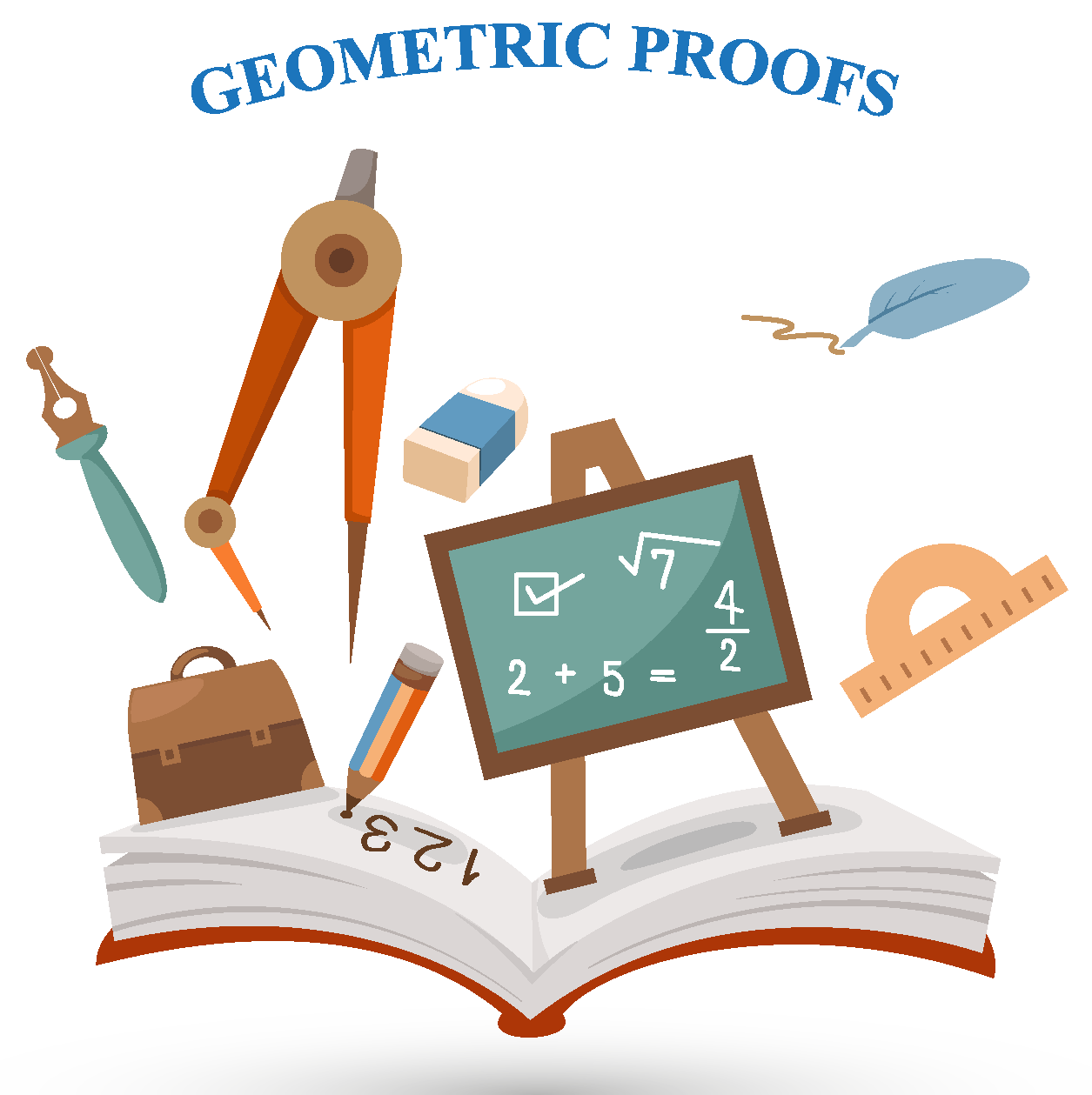In our study of geometry proofs, we will learn to do the same. We will learn how to construct a proof using only these axioms and postulates and using results that we have already proved earlier.

The foundation geometric proofs all exist only because of the truth of the various results and theorems.

Come, let us learn in detail about geometry proofs in this mini-lesson.

## Lesson Plan

 1 What are Geometric proofs? 2 Tips and Tricks 3 Solved Examples on Geometric proofs 4 Challenging Questions on Geometric proofs 5 Interactive Questions on Geometric proofs

## What Are Geometric Proofs?

A geometric proof is a deduction reached using known facts such as axioms, postulates, lemmas, etc. with a series of logical statements.

While proving any geometric proof statements are listed with the supporting reasons.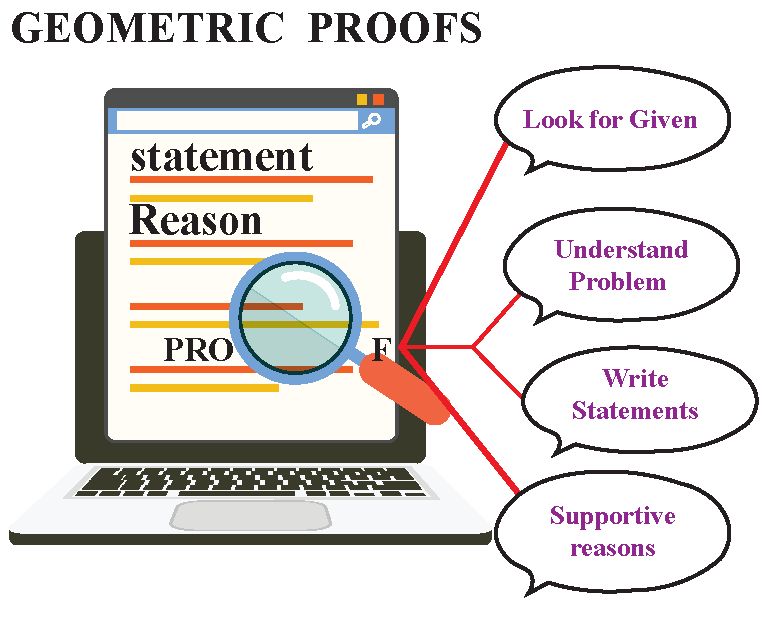## How Do You Write A Proof in Geometry?

Now that we know the importance of being thorough with the geometry proofs, now you can write the geometry proofs generally in two ways-

### 1. Paragraph proof

In this form, we write statements and reasons in the form of a paragraph.

let us see how to write Euclid's proof of Pythagoras theorem in a paragraph form.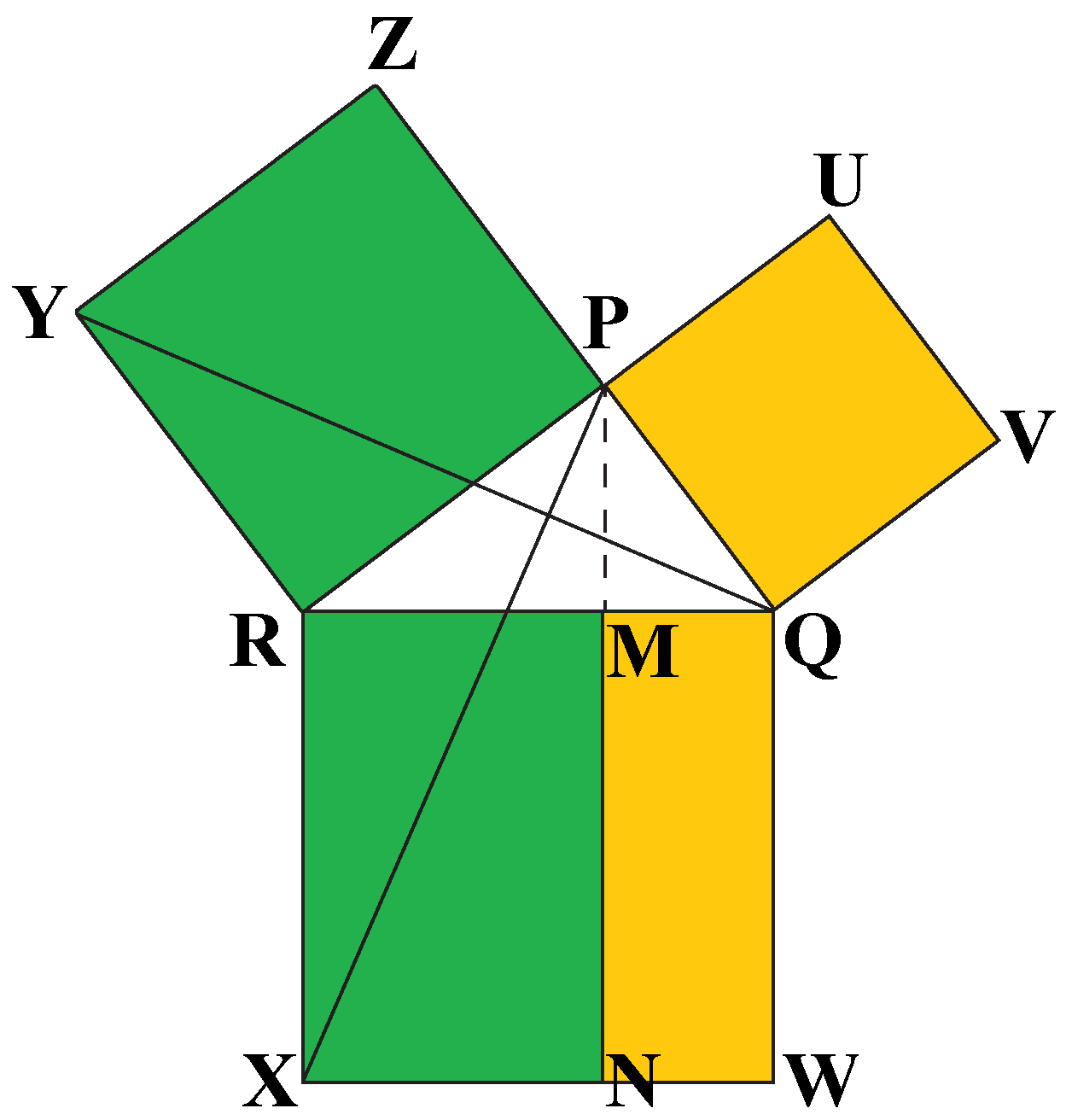Let $$PQR$$ be a right-angled triangle with a right $$\angle$$ $$QPR$$.

On each of the sides $$PQ$$, $$PR$$ and $$QR$$, squares are drawn, $$PQVU$$, $$PZYR$$, and $$RXWQ$$ respectively.

From $$P$$, draw a line parallel to $$RX$$ and $$QW$$ respectively.

Join $$PX$$ and $$QY$$, to form the $$\Delta$$ $$QRY$$ and $$\Delta$$ $$PRX$$.

$$\angle$$ $$QPR$$ and $$ZPR$$ are both right angles; therefore $$Z$$, $$P$$ and $$Q$$are collinear. Similarly for $$R$$, $$P$$ and $$U$$.

$$\angle$$ $$QRX$$ and $$\angle$$ $$PRY$$ are both right angles; therefore $$\angle$$ $$PRX$$ equals $$\angle$$ $$QRY$$ , since both are sum of $$90o$$ and $$\angle$$ ABC.

Since $$PR$$ is equal to $$RY$$ and $$RX$$ is equal to $$QR$$

$$\therefore \Delta PRX \cong \Delta QRY...(i)$$

Now, we know that when a rectangle and a triangle formed on a common base between the same parallels then area of triangle is half of the area of rectangle.

$$\therefore$$ $$Area\:of\:rectangle \:MNXR = 2 \times Area \:of \:Triangle\:QRY ...(ii)$$

$$\therefore$$ $$Area\: of \:Square \:PRYZ = 2 \times Area\:of \:Triangle\:PRX ...(iii)$$

Hence, from $$i$$, $$ii$$ and $$iii$$
$$Area\:of\:rectangle\:MNXR = Area\:of\:Square\:PRYZ ...(1)$$

Similarly, it can be shown that
$$Area\:of\:rectangle\:QWNM = Area\:of\:Square\:PQVU ...(2)$$

Adding $$1$$ and $$2$$ ,
$$PQ^2+ PR^2= XR\times XM + MN \times NQ$$

Since $$XR$$ = $$MN$$,

$$PQ^2+ PR^2= XR\times XM + XR \times NQ$$
$$PQ^2+ PR^2 = XR \times (XM + NQ)$$

Since $$QWXR$$ is a square

$$\therefore PQ^2+ PR^2 = QR \times QR = QR^2$$

Hence Proved.

### 2. Two-column proof

In this form, we write statements and reasons in the column.

For example, let us prove that If $$AX$$ and $$BY$$ bisects each other then $$\bigtriangleup AMB$$ $$\cong$$ $$\bigtriangleup XMY$$.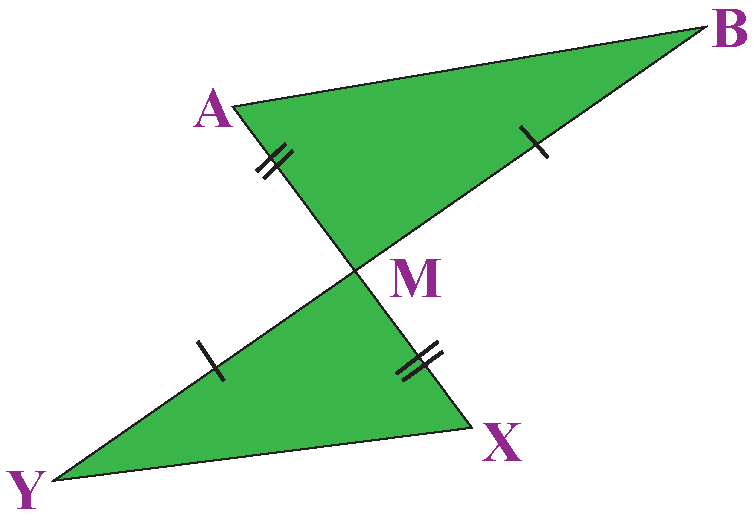Proof:

Statements Reasons

1. Line segments $$AX$$ and $$BY$$ bisecting each other.

2. $$AM$$ $$\equiv$$ $$XM$$ and $$BM$$ $$\equiv$$ $$YM$$

3. $$\angle$$ $$AMB$$ $$\equiv$$ $$\angle$$ $$XMY$$

4. \therefore  $$\bigtriangleup AMB$$ $$\cong$$ $$\bigtriangleup XMY$$

1. Given

2. When two line segments bisect each other then resulting segments are equal.

3. Vertically opposite angles are equal.

4. $$SAS$$ congruency axiom of triangles.Tips and Tricks

• Always figure out given information to find related derived results.
• Draw each part of the diagram separately.
• Relate "To Prove" statement with the given and diagram, it will help in writing the statements.

## Solved Examples

 Example 1

Prove that an equilateral triangle can be constructed on any line segment.

Solution

An equilateral triangle is a triangle in which all three sides are equal. Suppose that you have a segment $$XY$$: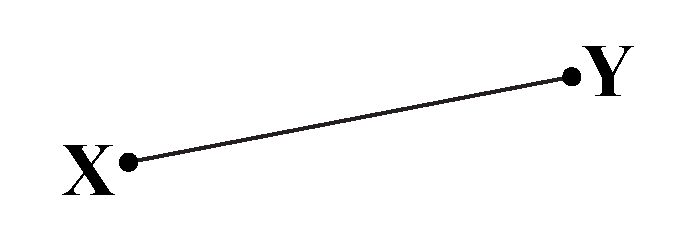You want to construct an equilateral triangle on $$XY$$. Euclid’s third postulate says that a circle can be constructed with any center and any radius. Now, construct a circle (a circular arc will do) with center $$X$$ and radius $$XY$$. Similarly, construct a circular arc with center $$Y$$ and radius $$XY$$. Suppose that the two circles (or circular arcs) intersect at $$Z$$. Join $$X$$ to$$Z$$ and $$Y$$ to $$Z$$.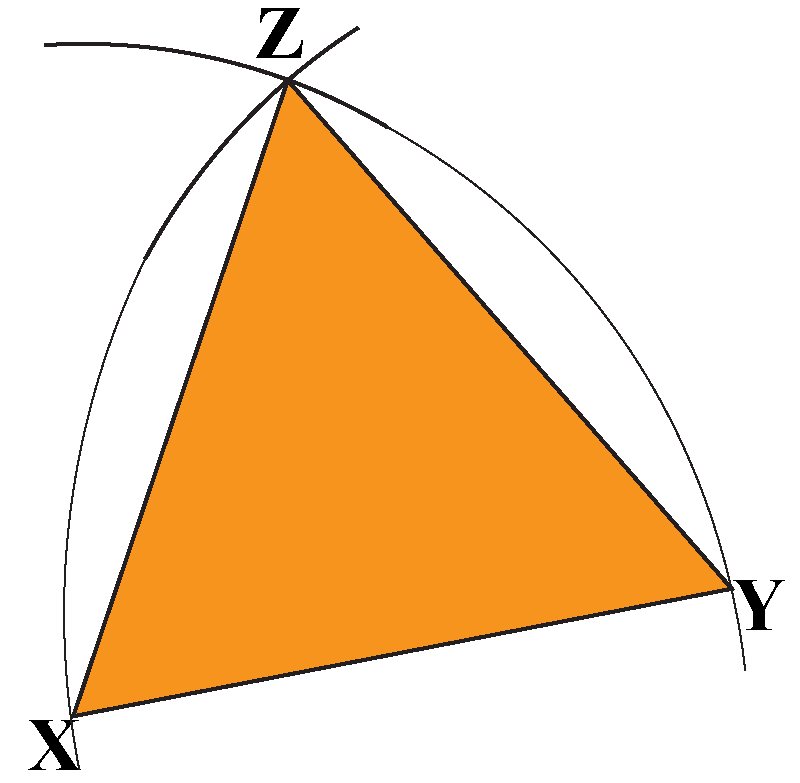Clearly, $$XY = XZ$$ (radii of the same circle) and $$XY = YZ$$ (radii of the same circle). Also, one of Euclid’s axioms says that things that are equal to the same thing are equal to one another. Thus,

$XY = YZ = ZX$

Thus, we have proved that an equilateral triangle can be constructed on any segment, and we have shown how to carry out that construction.

 $$\therefore$$ An equilateral triangle can be constructed on any line segment.
 Example 2

In the given figure, if $$AD$$ is the angle bisector of $$\angle$$ $$A$$ then prove that $$\angle$$ $$B$$ $$\equiv$$ $$\angle$$ $$C$$.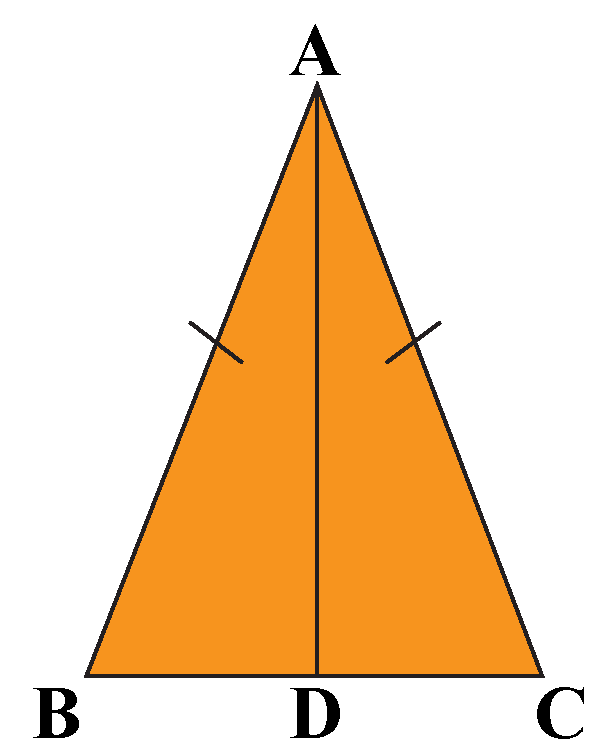Solution

Proof:
Given:
$$1.$$ Line segments $$AB$$ and $$AC$$ are equal.
$$2. AD$$ is the angle bisector of $$\angle$$ $$A$$
To prove:  $$\angle$$ $$B$$ $$\equiv$$ $$\angle$$ $$C$$

Proof: In $$\bigtriangleup BAD$$ and $$\bigtriangleup CAD$$

Statements Reasons

1. $$AB$$ $$\equiv$$ $$AC$$

2. $$\angle$$ $$BAD$$ $$\equiv$$ $$\angle$$ $$CAD$$

3. $$AD$$ $$\equiv$$ $$AD$$

4. $$\therefore$$  $$\bigtriangleup BAD$$ $$\cong$$ $$\bigtriangleup CAD$$

5. $$\therefore$$ $$\angle$$ $$B$$ $$\equiv$$ $$\angle$$ $$C$$

1. Given

2. $$AD$$ is the angle bisector of $$\angle$$ $$A$$

3. Common

4. $$SAS$$ congruency axiom of triangles.

5. CPCTC

 Hence proved.Challenging Questions

Write down the converse statement of the given statement and draw a figure using information.
"If a line is drawn parallel to one side of a triangle and it intersects the other two distinct points then it divides the two sides in the same ratio".

## Interactive Questions

Here are a few activities for you to practice. Select/Type your answer and click the "Check Answer" button to see the result.

## Let's Summarize

The mini-lesson targeted the fascinating concept of Geometric Proofs. The math journey around proofs starts with the statements and basic results that a student already knows, and goes on to creatively crafting a fresh concept in the young minds. Done in a way that not only it is relatable and easy to grasp, but also will stay with them forever. Here lies the magic with Cuemath.

At Cuemath, our team of math experts is dedicated to making learning fun for our favorite readers, the students!

Through an interactive and engaging learning-teaching-learning approach, the teachers explore all angles of a topic.

Be it worksheets, online classes, doubt sessions, or any other form of relation, it’s the logical thinking and smart learning approach that we, at Cuemath, believe in.

## FAQs on Geometric proofs

### 1. What are the geometric proofs?

A geometric proof is a deduction reached using known facts like Axioms, Postulates, Lemmas, etc. with a series of logical statements.

### 2. What jobs use geometry proofs?

Geometry is used in various fields by

• Designers
• Cartographer
• Mechanical Engineer etc.

### 3. What is a theorem?

The theorem is a general statement established to solve similar types of math problems.

### 4. Who is the father of geometry?

Euclid is the father of geometry.

### 5. What are the 3 types of proofs?

Three types of proofs are:

• Flow chart proof
• Paragraph proof
• Two-column proof

### 6. What are the 5 parts of a proof?

Five parts of proof are:

• Given Statement
• Diagram
• Proposition
• statements
• Reasons

### 7. What is flowchart proof?

In the flowchart proof reasons and statements are written in boxes.

Geometry
grade 9 | Questions Set 1
Geometry
grade 9 | Questions Set 2
Geometry
Geometry
More Important Topics
Numbers
Algebra
Geometry
Measurement
Money
Data
Trigonometry
Calculus
More Important Topics
Numbers
Algebra
Geometry
Measurement
Money
Data
Trigonometry
Calculus